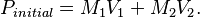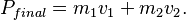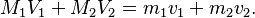# Momentum Conservation

One of the fundamental laws of the universe that any classical physics simulator, including Universe Sandbox, must follow in order to be able to reproduce realistic motions is the conservation of momentum. Momentum cannot be created nor destroyed, only transferred between objects, so the total momentum of a simulation must remain constant. This is especially important for collisions, in which momentum is exchanged between the colliding objects as they alter each other's velocities.

A similar fundamental law is the conservation of angular momentum: the total angular momentum of a simulation must also remain constant.

## Related Properties & Settings

### Properties

• The amount of momentum that is lost in the simulation due to the loss of mass (see Limitations) can be seen in the Stats menu in the bottom bar.

## Models

Consider an object with mass M1 and velocity V1 colliding with another object with mass M2 and velocity V2. Before the collision, the total momentum of this simulation is$P_{initial} = M_1 V_1 + M_2 V_2.$

After the collision, the two objects may have new velocities and even new masses, if some of the mass transferred from one body to the other during the collision. Suppose that after the collision, object 1 has mass m1 and velocity v1, and object 2 has mass m2 and velocity v2. Then the total momentum of the simulation after the collision is$P_{final} = m_1 v_1 + m_2 v_2.$

According to the law of conservation of momentum, momentum cannot be created or destroyed, so the total momentum of the simulation must be the same before and after the collision:$M_1 V_1 + M_2 V_2 = m_1 v_1 + m_2 v_2.$

Universe Sandbox uses this equation to help calculate what the new velocities, v1 and v2, of the objects will be after the collision.

## Limitations

Occasionally, mass is lost from the simulation for performance reasons. This lost mass carries with it some momentum, which is therefore also lost from the simulation.